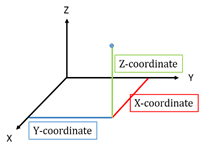# Vector and 3d geometry jee mains notes and Previous year Questions

Syllabus- Coordinates of a point in space, distance between two points, section formula, direction ratios and direction cosines, angle between two intersecting lines. Skew lines, the shortest distance between them and its equation. Equations of a line and a plane in different forms, intersection of a line and a plane, coplanar lines.

Vectors and scalars, addition of vectors, components of a vector in two dimensions and three dimensional space, scalar and vector products, scalar and vector triple product.

Important Topics for JEE Main 2020 in Mathematics

In the Mathematics section of JEE Main, there is an even distribution of marks for all the chapters. There are 15 chapters and all are equally important chapters for JEE Main. So, you can prioritise focusing on particular chapters which are easy and the chapters in which you have a good grip.

Below is a list of scoring chapters and important chapters for JEE Main in Mathematics:

• Sequence & Series
• Determinants and Matrices
• Sets and relations
• Mathematical Reasoning
• Statistics & Probability
• Vector & 3D
• Straight line
• Circle
• Definite Integral
• Indefinite Integral
• Application of Derivatives
• Differential equation
• Application of Integrals
• Trigonometric Ratios

MATHS EASY AND IMPORTANT CHAPTERS ( JEE MAINS 2020 )

1. Statistics- 4 marks
2. Height and Distance- 4 marks
3. Mathematical Reasonong- 4marks
4. Matrices and Determinant- 8 marks
5. Sets,Relation and function – 8 marks
6. Vector and 3D– 8 marks
8. Area – 4 marks
9. Limit , continuity and derivability–8 marks
10. Conic section – 12 marks

1.Faisal says:
2.Saurabh kumar says: